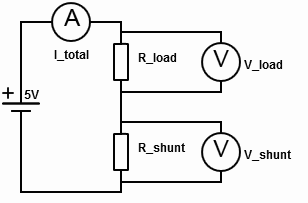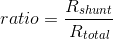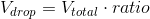# Shunt

## How does a shunt works?

Short:
A shunt is a very small resistor that is used to measure current. The resistor is added in series to the load and the voltage drop is measured. From the voltage drop and the value of the resistor the current can be calculated.

In Detail:
The best way to get a better understanding is to have a look at the principle of voltage divider.In a voltage divider the current is always the same.-> Means we can really use a second resistor to find out the current that is flowing through the load.

Every resistor has a voltage drop that has the ratio of the resistor to the total resistance.-> This tells us that we have to choose the resistor as small as possible so that we don’t drag to much voltage from the load.

This table displays how the resistance value of the R_shunt drains power if it is too high. Also you can see how small the voltage drop gets if the resistance is to low, what makes it hard to measure.

I (total) U (total) R_load R_shunt U (load) U (shunt) P (lost)
0,25A 5V 10 10 2.5V 2.5V 0.625W
0,25A 5V 19 1 4.75V 0.25V 0.0625W
0,25A 5V 19.9 0.1 4.975V 0.025V 0.00625W

Arduino

## Usages

• Energy generation
• Energy consumption
• Battery charging

## Alternatives

Hall-Effect current sensing